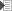# 3.4 Decibels

## Ratios and exponents

The decibel, symbol dB, is a logarithmic scale initially introduced as the Bel, but the deciBel seemed more practical (1 B = 10 dB). The dB is not a unit, like, for instance, the Volt. Rather, it describes the magnitude ratio of two numbers along a logarithmic scale. Decibels express the exponent of the ratio of two numbers, one of which is the reference. This looks very complicated, and unfortunately, it is. But decibels are an excellent way to express number that are very far apart. To complicate matters even more, there are many different standard references, indicated by a suffix trailing the dB symbol, and signal levels and power levels require their own conversion equations.

## References used in audio

• Signal level
• dBv: Volt. Reference is set as 0 dB = 1 V.
• dBu: Unloaded. This is the standard reference to specify signal levels in professional audio. Set as 0 dBu = .775 V.
• Power level

## Silence

So when 0 dB equals 1 V or .775 V or 1 W, then what is silence? This is another strange thing about decibels. Silence equals minus infinity, or -∞

## Power levels

Due to of the characteristics of our hearing, sound pressure levels (SPL) are expressed in decibels, symbol dB(SPL). This is in such a way that adding 10 dB means multiplying the sound pressure (and the necessary amplifier power) by 10. Consequently, adding 20 dB means multiplication by 100, 30 dB is times 1,000 etc. As said before, decibels indicate a ratio (P1/P2), so there's always need for a reference level. For instance, when measuring absolute sound pressure level in a room, the hearing threshold is used as a reference, and set to 0 dB. When calculating the maximum SPL of a loudspeaker, the reference level would be the efficiency rating (sometimes called "reference efficiency"), which in turn references to the hearing threshold. In mathematical terms (told you so, we don't stick to the basics):

 PdB = PdBref + 10 · 10log ( P ) Reference in dB P = 10 ( ( PdB - dBref ) / 10 ) Reference in dB PdB = 10 · 10log ( P / Pref ) Reference in watts P = Pref · 10 ( PdB / 10 ) Reference in watts

Example 1 (1st equation): you have a speaker with a reference efficiency of 96 dB (specified at 1 W at 1 m), and a maximum input power of 250 Watts. The maximum achievable SPL is: SPL = 96 + 10 · 10log ( P ) = 96 + 10 · 10log ( 250 ) = 96 + 24 = 120 dB. So, the speaker produces 96 dB at 1 W of input power. This is the reference. We want to multiply the power by 250. This adds 24 dB to the total SPL, giving 120 dB.

Example 2 (3rd equation): an amplifier's maximum output power is 300 W. You need more power and decide to purchase a 500 W amplifier. That way you have 500 / 300 = 1.67 times as much power available. SPL will increase by: SPL = 10 · 10log ( 500 / 300 ) = 2.2 dB.

Please refer to the appendixfor a list of quick dB(SPL) to power level conversions.

## Signal levels

It was mentioned before: power equals voltage square divided by resistance (P = V2/R). Because of the square in the equation, signal levels, which are voltages, require another method of determining dBs. With signal levels, a rise of 20 dB (instead of 10) is multiplication by ten.

 VdB = 20 · 10log ( V / Vref ) Reference in volts V = Vref · 10 ( VdB / 20 ) Reference in volts VdB = VdBref + 20 · 10log ( V ) Reference in dB V = 10 ( ( VdB - dBref ) / 20) Reference in dB

Example 1 (2nd equation): An effects pedal accepts a signal level of -10 dBu. This is a very common value and is called "line" level. Converting this value to a voltage gives: V = .775 · 10 ( -10 / 20 ) = .775 · .316 = .25 V

Example 2 (2nd equation): A rackmount effects unit accepts a signal level of +4 dBu. Again, this is a very common value, sometimes called "studio" or "professional" level. In Volts this is: V = .775 · 10 ( 4 / 20 ) = .775 · 1.585 = 1.23 V

Example 3 (1st equation): When measuring a test sine wave with a digital voltmeter, the display reads 1.00 Volts. Determining the signal level in dBu yields VdB = 20 · 10log ( 1.00 / .775 ) = 2.21 dBu

© Joris van den Heuvel 2001-2009